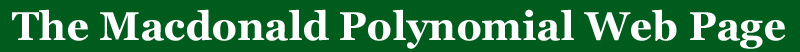BACK TO:Cyclage Posets

These diagrams can be used to read off the Macdonald polynomials in the case whenis two rows or two columns. The cyclage poset has other combinatorial uses so a black and white version is available. There is a file (READMECP.tex, READMECP.ps, READMECP.pdf) to accompany the color versions that explains the statistics on standard tableaux.

### LaTEX versions

• Requires: tabmac.sty (Glenn Tesler)
The color is done with Textures commands
• n=4 color for=(2,2)
• n=5 color for=(2,2,1)
• n=6 color for=(2,2,2)
• n=4 b&w
• n=5 b&w
• n=6 b&w
• ### postscript versions

• n=4 color for=(2,2)
• n=5 color for=(2,2,1)
• n=6 color for=(2,2,2)
• n=4 b&w
• n=5 b&w
• n=6 b&w
• n=4 color for=(2,2)
• n=5 color for=(2,2,1)
• n=6 color for=(2,2,2)